Question

# Two thin lenses of focal lengths 86 mm and -92 mm are placed in contact. What...

Two thin lenses of focal lengths 86 mm and -92 mm are placed in contact. What power must a third lens have so that when the three are in contact the combination has a focal length of -45 mm?

ANS...... diopters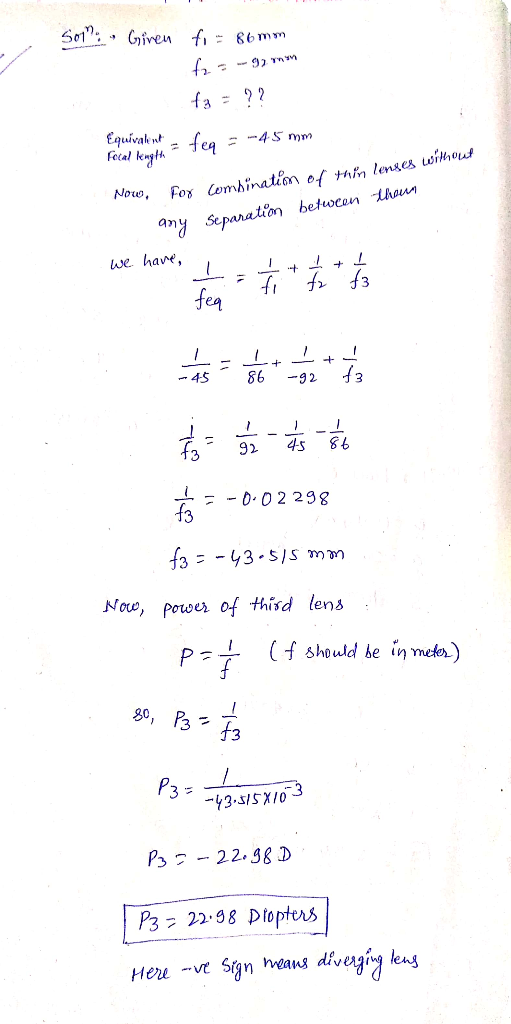#### Earn Coins

Coins can be redeemed for fabulous gifts.

Similar Homework Help Questions
• ### 10. Two lenses are in contact. One of the lenses has a focal length of +10.0cm...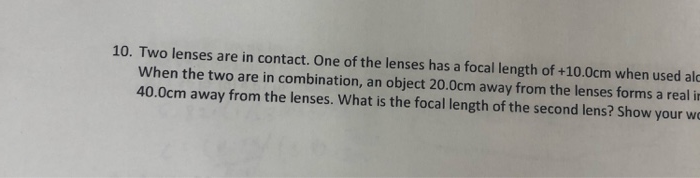10. Two lenses are in contact. One of the lenses has a focal length of +10.0cm when used ale When the two are in combination, an object 20.0cm away from the lenses forms a reali 40.0cm away from the lenses. What is the focal length of the second lens? Show your w 9. One lens has a focal length of +15.0 cm. A second lens of focal length +20.0cm is placed in contact with the first lens. What is the...

• ### When two thin lenses are in contact, the combination may still be considered as a thin...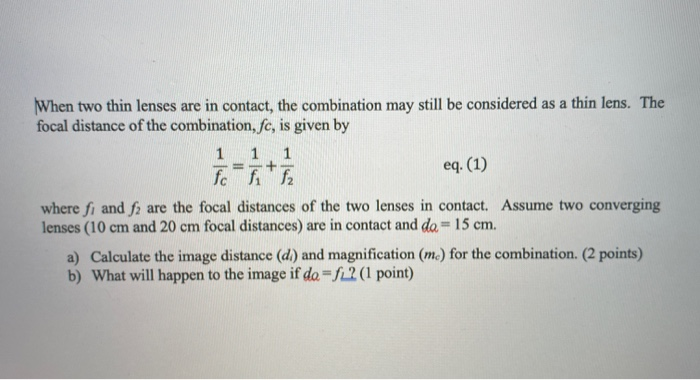When two thin lenses are in contact, the combination may still be considered as a thin lens. The focal distance of the combination, fc, is given by 1 1 1 + eq. (1) fcff2 where fi and f are the focal distances of the two lenses in contact. Assume two converging lenses (10 cm and 20 cm focal distances) are in contact and da = 15 cm. a) Calculate the image distance (d) and magnification (m.) for the combination. (2...

• ### Two positive thin lenses are separated by a distance of d. The focal lengths of the...

Two positive thin lenses are separated by a distance of d. The focal lengths of the lenses are f_1 = 10 cm and f_2 = 20 cm. The desired throw of the system, the object to image distance, is T = 80 cm and the desired magnification is M = -1.1. a) Use what you know about the conjugate matrix for a two lens system to solve to the distance between the lenses. Express your answer in cm. b) Where...

• ### Two thin lenses with focal lengths of magnitude 18.0 cm, the first diverging and the second...

Two thin lenses with focal lengths of magnitude 18.0 cm, the first diverging and the second converging, are placed 11.00 cm apart. An object 3.70 mm tall is placed 4.75 cm to the left of the first (diverging) lens. (a) Where is the image formed by the first lens located? (b) How far from the object is the final image formed? (c) Is the final image real or virtual? (d) What is the height of the final image? (e) Draw...

• ### 2. An object is placed in front of two thin symmetrical convex lenses with focal lengths...2. An object is placed in front of two thin symmetrical convex lenses with focal lengths of F1 +10 cm and F25 cm, with a lens separation of D-42 cm. The object is 15.0 cm from lens 1. Where is the final image of the object and what is its magnification? (10 points) [Please use reverse side if you need more room. Circle the correct descriptors: real virtual enlarged reduced erect inverted Answer: X final = ML =

• ### Two converging lenses, with focal lengths of f1 = 10.0 cm and f2 = 15.0 cm,...

Two converging lenses, with focal lengths of f1 = 10.0 cm and f2 = 15.0 cm, respectively, are placed 40.0 cm apart. An object is placed 60.0 cm in front of the first lens. (a) Calculate the position, with respect to the second lens, of the final image formed by the combination of the two lenses. (b) Calculate the overall magnification of the final image formed by the combination of the two lenses.

• ### A person sees clearly wearing eyeglasses that have a power of -3.00 diopters when the lenses...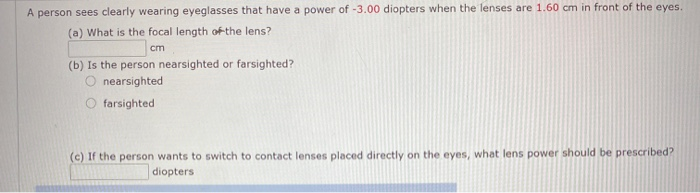A person sees clearly wearing eyeglasses that have a power of -3.00 diopters when the lenses are 1.60 cm in front of the eyes. (a) What is the focal length of the lens? cm (b) is the person nearsighted or farsighted? O nearsighted farsighted (e) If the person wants to switch to contact lenses placed directly on the eyes, what lens power should be prescribed? diopters

• ### Two identical converging lenses of focal length 100 mm are placed 400 mm apart, and an...

Two identical converging lenses of focal length 100 mm are placed 400 mm apart, and an object 40 mm tall is placed 230 mm to the left of the left lens. Part B Is the image formed by the right lens inverted or upright?

• ### A person sees clearly wearing eyeglasses that have a power of -3.00 diopters when the lenses...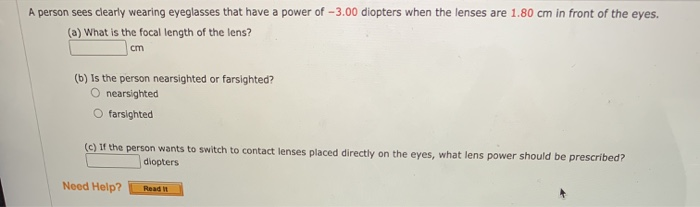A person sees clearly wearing eyeglasses that have a power of -3.00 diopters when the lenses are 1.80 cm in front of the eyes. (a) What is the focal length of the lens? cm (b) is the person nearsighted or farsighted? O nearsighted farsighted (C) If the person wants to switch to contact lenses placed directly on the eyes, what lens power should be prescribed? diopters Need Help? Read it

• ### A camera is supplied with two interchangeable lenses, whose focal lengths are 38.0 and 110.0 mm....

A camera is supplied with two interchangeable lenses, whose focal lengths are 38.0 and 110.0 mm. A woman whose height is 1.77 m stands 8.10 m in front of the camera. What is the height (including sign) of her image on the image sensor, as produced by (a) the 38.0-mm lens and (b) the 110.0-mm lens?